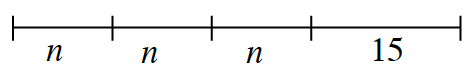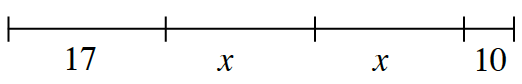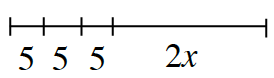### Home > CC1 > Chapter 5 > Lesson 5.3.4 > Problem5-104

5-104.

Write an algebraic expression to represent the length of each segment shown below.

1.$n + n + n + 15 or 3n + 15$

2.$17 + x + x + 10$. Can you find a simpler
way to write this expression?

3.• See parts (a) and (b).